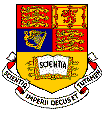Imperial College London

Department of Electrical & Electronic Engineering

Electrical & Electronic Eng. (EEE)        BEng, MEng 2nd Year
Information Systems Eng. (ISE)
BEng, MEng 2nd Year

E2.5 Signals & Linear Systems (Spring 2011)

##Objectives

The course is designed to provide the fundamental concepts in signals and systems. By the end of the course, students should be able to use signal transforms, system convolution and describe linear operations on these.

Syllabus

We draw a distinction between the fundamentals of signal modelling in time and frequency domains, and indicate the signficance of alternative descriptions. The basic concepts of Fourier series, Fourier transforms, Laplace transforms and related areas are developed. The idea of convolution for linear time-variant systems are introduced and expanded on from a range of perspectives. The transfer function for continuous and discrete tiem systems is used in this context. Stability is duscussed with respect to the pole locations. Some elements of statistical signal description are introduced as signal comparision methods. The Discrete Fourier Transform is discussed as a z-transform evaluation and its consequences examined. Some basic filtering operatings for both continuous and discrete signals are developed.

Textbook & Key References

Matlab Licence

This course includes the use of Matlab for tutorial problems. Two Matlab tutorial sessions will be given at the beginning of the course. It is important that you have a copy of Matlab installed and properly licensed under Imperial College's Licensing Scheme. You can find the instruction about how to obtain Matlab here (Imperial login required). Since you are a full-time member of the College, if you wish to install Matlab on a personally owned system, please complete a licence form available here. For installation instructions, please click here.

Matlab is also installed on ALL machines in the Department.

Lecture Notes & Problem Sheets

 Week Notes Problem Classes 1 Lecture 1 - Introduction to Signals Lecture 2 - Introduction to Systems Matlab Tutorial 1 Problem Sheet 1 (Solutions) 2 Lecture 3 - Zero-input Responses Lecture 4 - Zero-state Responses Matlab Tutorial 2 Problem Sheet 2 (Solutions) 3 Lecture 5 - Convolution Lecture 6 - Laplace Transform 4 Lecture 7 - More on Laplace Transform Lecture 8 - Frequency Response 5 Lecture 9 - Poles, Zeros & Filters Lecture 10 - Fourier Transform 6 Lecture 11 - More Fourier Transform Lecture 12 - Windowing Effects 7 Lecture 13 - Sampling & Discrete Signals 8 Lecture 14 - Discrete Fourier Transforms Lecture 15 - z-transform Problem Sheet 8 (Solutions) 9 Lecture 16 - More z-transform Lecture 17 - Digital Filters Problem Sheet 9 (Solutions)

Sample & Past Papers

Note that as from 2011, all 2nd year papers contain only COMPULSORY questions.